#### 期刊菜单

Numerical Simulation of Flow around a Near Streamlined Beam Section

Abstract: Taking the nearly streamlined box girder section commonly used in long-span bridges as the re-search object, the flow field characteristics of the section are analyzed through the numerical si-mulation method of computational fluid dynamics (CFD). And use the Fluent software to numeri-cally simulate the flow around the cross-section of the nearly streamlined main girder, and obtain the static mean aerodynamic force coefficient under the wind axis coordinate within the range of −6˚~+6˚ wind attack angle, and the post-processing software processes to obtain ±5˚ Schematic diagram of flow field distribution of bridge section under wind attack angle (including pressure distribution diagram, velocity distribution diagram and streamline diagram). The analysis results show that the wind attack angle is the main factor affecting the mean aerodynamic force coefficient of the beam section, and as the wind attack angle increases, the drag coefficient first decreases and then increases, the lift coefficient gradually increases, and the torque coefficient gradually decreases.

1. 引言

CFD是近年来快速发展的一种研究方法，该方法基于流体动力学的基本理论，借助计算机技术来模拟桥梁结构的流场分布以及风–结构的相互作用。目前CFD技术可以按实际的风环境进行仿真和模拟，能直观的表现出桥梁结构周围的流场特征 。在桥梁模型进行风洞试验前，可以通过CFD技术对其进行数值模拟，为试验方案的可行性进行预测，避免因试验方案错误造成资源浪费 。利用计算流体力学软件Fluent，对某近流线型主梁断面绕流进行仿真模拟，计算静力三分力系数，并用后处理软件Tecplot得到±5˚攻角下箱梁断面的压强、速度分布图和流线图。

2. 静力三分力系数

$P+\rho {U}^{2}=常数$ (1)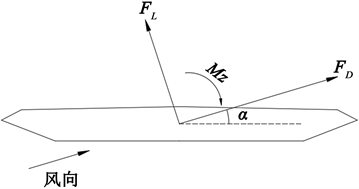Figure 1. Static mean aerodynamic force coefficient in wind axis coordinates

3. 主梁断面绕流的数值模拟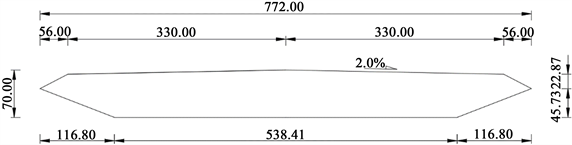Figure 2. Dimensions of the main beam section after scaled down (unit: mm)

3.1. 计算域的选取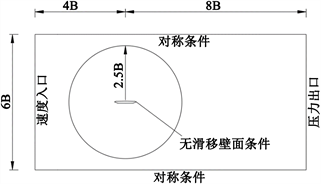Figure 3. Schematic diagram of computational domain

3.2. 湍流模型及边界条件设置

3.3. 网格划分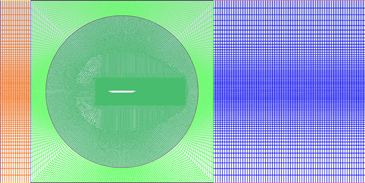Figure 4. Schematic diagram of the overall grid of the flow field around a streamlined beam sectionFigure 5. Schematic diagram of the local grid of the flow field around a streamlined beam section

4. 计算结果

4.1. 静力三分力系数模拟结果

1) 风攻角为影响桥梁结构静力三分力系数的影响因素，且对升力系数影响最明显；

2) 阻力系数随着风攻角的增加先减小后增加：在风攻角由−6˚变化到0˚过程中，阻力系数变化趋势较大，而风攻角由0˚变化到+6˚过程中，阻力系数变化相对平缓；阻力系数在风攻角为0˚时为0.2512；

3) 风攻角由−6˚增加到+6˚过程中，升力系数逐渐增加；

4) 风攻角由−6˚增加到+6˚过程中，扭矩系数逐渐减小，且基本为线性关系；当风攻角为负值时，扭矩系数为正值；当风攻角为正数时，扭矩系数为负值。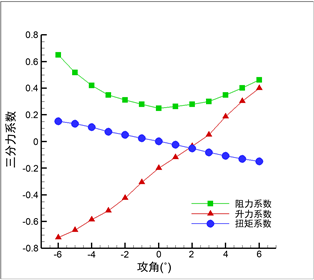Figure 6. Schematic diagram of the mean aerodynamic force coefficient of a streamlined section changing with the angle of attack of the wind

4.2. 流场分布示意图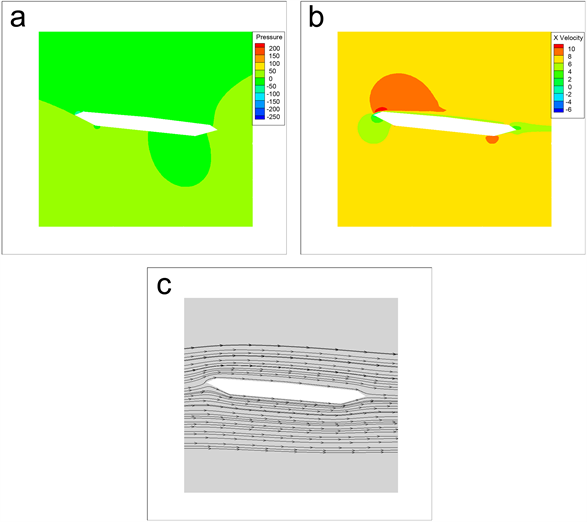Figure 7. Schematic diagram of flow field distribution of nearly streamlined section at +5˚ wind attack angle. (a) Pressure distribution map; (b) Flow rate graph; (c) Streamline diagram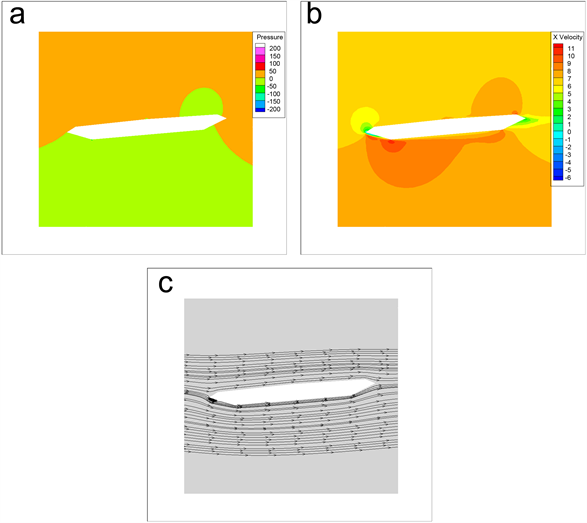Figure 8. Schematic diagram of flow field distribution of nearly streamlined section at −5˚ wind attack angle. (a) Pressure distribution map; (b) Flow rate graph; (c) Streamline diagram

1) 对压强分布图而言，当来流风速流经截面时，在截面的迎风面出现了较大的高压区，而在截面的顶部和底部都出现了负压区；

2) 对流速图而言，对气流受到阻碍速度明显减小；

3) 对流线图而言，断面尾流区没有出现涡脱现象；

4) 能直观显示主梁断面周围的流场特性。

5. 结语

1) 通过合理的建模和设置参数，Fluent能够获得该断面的三分力系数关于风攻角的变化规律；

2) 风攻角为影响桥梁结构静力三分力系数的影响因素，且对升力系数影响最明显；

3) 随着风攻角的增大，阻力系数先减小后增加，升力系数逐渐增加，扭矩系数逐渐减小；

4) 该仿真结果可为实际风洞试验方案提供参考。

  曹曙阳. 计算流体力学在风工程中的应用[C]//中国土木工程学会桥梁与结构工程分会风工程委员会. 第十四届全国结构风工程学术会议论文集(上册). 2009: 15.  陈廷君. 采用CFD方法的钝体桥梁断面风阻系数研究[D]: [硕士学位论文]. 重庆: 重庆交通大学, 2013.  吴瑾, 夏逸鸣. 土木工程结构抗风设计[M]. 北京: 科学出版社, 2007: 124.  陈艾荣. JTG-T. 3360-01-2018公路桥梁抗风设计规范[S]. 北京: 人民交通出版社, 2018.  原学明. 桥梁典型截面静风系数数值模拟研究[D]: [硕士学位论文]. 大连: 大连理工大学, 2008.  李薇, 胡兆同, 李加武, 张茜. 桥梁断面三分力系数数值模拟[J]. 公路交通科技, 2012, 29(5): 37-40+45.  谭红霞, 陈政清. CFD在桥梁断面静力三分力系数计算中的应用[J]. 工程力学, 2009, 26(11): 68-72.  张志田, 张显雄, 陈政清. 桥梁气动力CFD模拟中湍流模型的应用现状[J]. 工程力学, 2016, 33(6): 1-8.  安里鹏, 李德建, 王元礼, 袁鹏. 基于CFD方法的高墩大跨箱梁桥静风力系数参数影响研究[J]. 应用力学学报, 2016, 33(1): 143-149+188.  王锋. 基于CFD对大跨度连续桥梁抗风性能分析[J]. 公路工程, 2018, 43(3): 83-86+167.  汪家继, 樊健生, 聂建国, 杨小刚, 李连友. 大跨度桥梁箱梁的三分力系数识别研究[J]. 工程力学, 2016, 33(1): 95-104.  肖鸿治. 高速铁路典型桥梁断面静力三分力系数研究[D]: [硕士学位论文]. 北京: 北京交通大学, 2016.  项海帆, 葛耀君. 现代桥梁抗风理论及其应用[J]. 力学与实践, 2007(1): 1-13.  应旭永. 大跨度桥梁颤振性能的数值模拟研究[D]: [博士学位论文]. 大连: 大连理工大学, 2017.